# Excel VLOOKUP Formula Examples – Including how to create dynamic vlookup formulas

Last updated on January 16, 2013 By

In part 1 of this series we took a look at VLOOKUP formula basics. If you want to learn how a VLOOKUP formula works, or know someone else who is struggling with VLOOKUPs, check out the first article “Discover a simple way to understand how VLOOKUP formulas work in Excel“, and come back to this article.

Here in part 2, we have a 14 minute video that explains three different ways to write the VLOOKUP formula for exact matches. If you have never used the COLUMNS function or MATCHfunction with VLOOKUP, you are in for a treat because you will find out how to turn the standard VLOOKUP into a dynamic formula. As usual I have included a sample workbook with a table of fictional employee data for you to download and follow along with the examples.

Image Credits:
CGI waves courtesy of “gerard79“.
Abstract circle shape courtesy of “iprole“.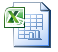Note: the sample workbook has been tested on Excel 2010 (32-bit, English language version) and you may get different results in other version of Excel. If you find that you can’t use this workbook on your version of Excel, please let us know using the comment section below.

The sample download workbook contains slightly different cell references compared to the workbook used in the video, but the data itself is identical and provides a convenient way for you to practise your VLOOKUP formulas.

VLOOKUP Tutorial Video Part 2

Watch our 14 minute video to help you understand three different ways of writing the VLOOKUP formula for exact matches. Click on the video below to play it and listen to my audio commentary.

## Video Transcript

Note: The video was transcribed by SpeechPad.com and then edited into the form you see below.

We’re going to have a look at three different ways to write VLOOKUP formulas. One is a straightforward, simple approach, the second is using the columns function, and the third is using the MATCH function.

Here we have an employee LOOKUP table with the employee ID on the left. You see we’ve got employee full name, SSN, department, start date, and earnings for each of these employees.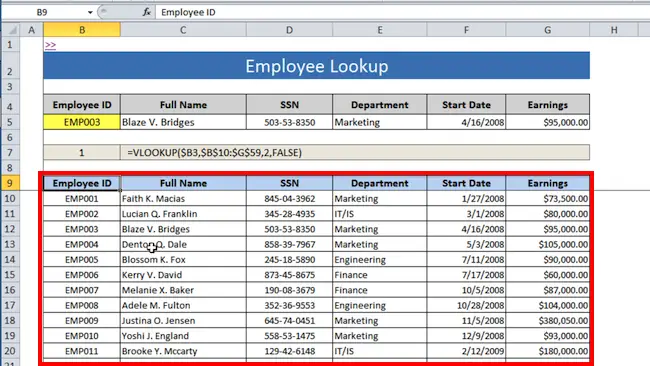along.

What we’re going to do is use a VLOOKUP formula to populate this top table, so that whenever we change the data validation drop-down, say we change it from 3 to 2, we shall see the corresponding line pulled out at the top.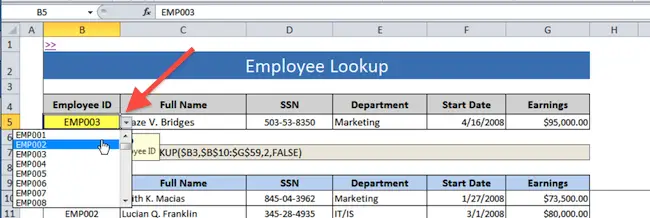Change the drop-down selection from EMP003 to EMP002 and watch the formula output

update

None of the names here are real people, they’re all computer-generated names. So if you see someone’s name that you recognize, it isn’t that person.

By the way, if you want to learn more about data validation, you should check out my book “Power Tips for Excel.” That takes you through some data validation tips and tricks.### What we will cover in this video

Now I’m going to take you through three different ways of writing the VLOOKUP formula.

1. Simple way – type in numbers for column index number

2. COLUMN function – use the COLUMN function to specify column index number

3. MATCH function – use the MATCH function to specify column index number

The first is the standard approach, the second uses the COLUMN function, and the third uses the MATCH function. While the first way is the simple way, it is actually not the most efficient in this case. We’ll go through method one, then we’ll check out the column method and the match method which in my opinion are usually more useful.

### VLOOKUP Method One – Type in numbers for column index number (not dynamic)

The simple method is to use the VLOOKUP formula without any other functions. Go to cell C5 and we will set up the VLOOKUP to pull up “Full Name” using the employee ID. In the formula bar, type =VLOOKUP, then type out the full VLOOKUP formula. When I click on E5 I also press the F4 key (three times) to lock this to absolute references so it doesn’t move from column B (\$B5).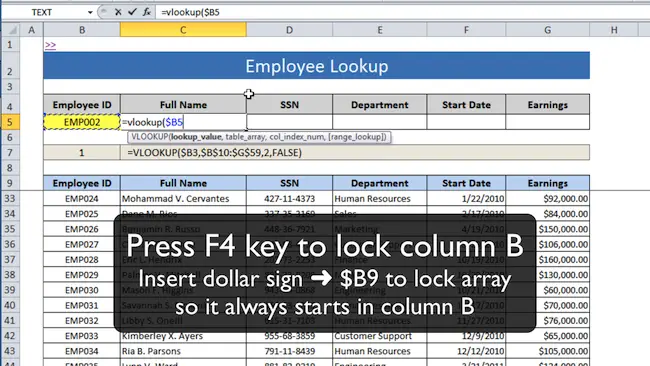Press the F4 key to change cell reference style to absolute column reference (\$B5 where

\$ means column B stays fixed or “absolute”)

The table array is down here, but I’m not going to select the column headings. I will just select the data. Again I press the F4 key once to lock it to absolute reference (\$B\$10:\$G\$59).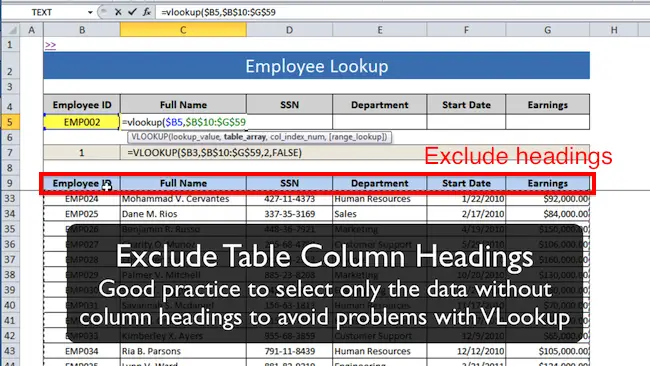It is usually a good idea to exclude column headings when you specify the data range for

Next I enter the column index, which is 2 for “Full Name”. Finally I type False, to specify that I want an exact match.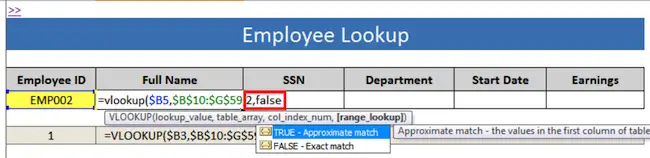Complete the VLOOKUP formula by specifying 2 for “col_index_num” and FALSE for

“range_lookup” type, meaning 2nd column in table_array and FALSE to return an exact

match.

Press the Enter key, and you’ll notice that employee EMP002 gets pulled up, Lucian Franklin. And what I should be able to do now is copy that across into each of these cells (D7:G7). What I’ll do is go Control + C for copy, and Control + Alt + V for paste special because I don’t want to overwrite the formatting, so I’m going to select paste formulas.

Now, you see it’s just put the full name in each of these boxes, which we don’t want.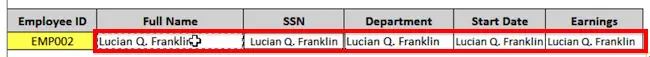This is what happens after copying the formula right. Excel does not know that you want to

look up different values, so it looks up the same value from column 2 in every cell.

Instead, I need to go up here, and change the column index number from a 2 to a 3. Press tab, and then for Department, I have to change it from a 2 to a 4. Press tab again to move right. And then in Start Date, I need that to be a 5. Press tab. And in Earnings, I need that to be a 6. (See screenshot below)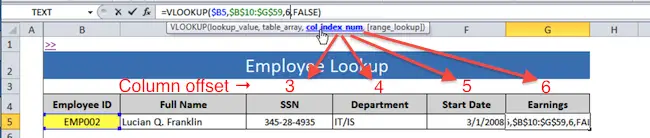We need to change the formula in each cell to update the col_index_num. Each cell now

has the correct VLOOKUP column offset, and returns the correct lookup value.

So now we have the LOOKUP table completed using the simple approach. But it does mean that you have to manually enter 2, 3, 4, 5, and 6 as the column offset because when you copy and paste the VLOOKUP formula the col_index_num does not automatically update for you.

## VLOOKUP Method Two – Use the COLUMN function to specify column index number (semi-dynamic)

Method two uses the columns function, which you can see here. So the formula is going to end up like this, it’s going to be a VLOOKUP with the standard parameters. But it’s also going to use the function COLUMNS with an array, and I explain that later, with a FALSE at the end for an exact match.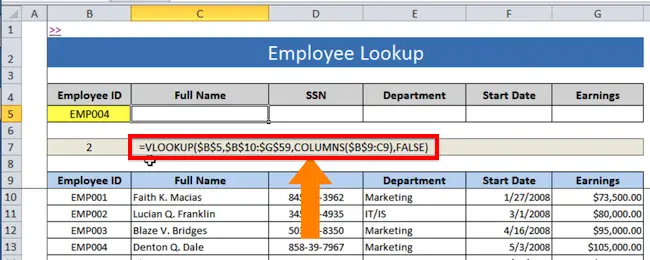Method 2 – VLOOKUP with the COLUMN function

Go to cell C5 and type =VLOOKUP(. Select cell B5 as the lookup_value, and press F4 to lock the cell reference to \$B\$5. That means when it is copied across, it will always reference cell B5.

For the table_array, I will select the data excluding the column headings (as before, this is good practice when writing VLOOKUP formulas). Press Control + Shift + Right arrow, Control + Shift + Down arrow, and that will select all the data in the table (this works because there are no empty data cells). Press the F4 key to lock that to absolute references.

And now, for the column index number, for “Full Name”, I want it to come out with a 2. And what the function COLUMNS does is it counts the number of columns in a particular array, where an array is just a group of cells on the worksheet.

We want the array to start at B9 and go up to C9. I’m going to lock the range reference to the column B, by inserting a dollar sign (\$B9:C9). And so when I copy this across, you’ll that the first cell in the range reference stays in column B while the second cell in the range reference moves from column C to column D to column E etc. depending on how far I copy across (e.g. \$B9:E9)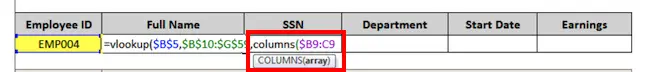Specify the array used inside the COLUMNS function as \$B:C9

The last argument in the VLOOKUP formula is the range_lookup, and I set that to FALSE for an exact match.

So for employee EMP004 it has found “Denton Q Dale”. And now I’m going to copy the VLOOKUP formula, using Control + Alt + V to copy and paste special formulas only, so I don’t overwrite the existing cell formats.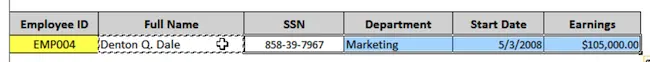When the VLOOKUP formula is copied across, the COLUMNS(\$B9:C9) function

dynamically takes care of the col_index_num so there is no need to manually update it for

every cell.

Now to explain what happened. The COLUMNS function looks at the range of cells you give it, and counts the number of columns in that range. As you copy the formula across, you notice that the range stays fixed on column B, so it stays at \$B9, but as I copy it across the second part of the range changes to C9, then D9, then E9, then F9 and finally G9 depending on how far to the right I copy.

So in cell G5, the COLUMNS function looks at the range \$B9 to G9, and counts how many columns are there. So that would be one column, two columns, three columns, four columns, five columns, six columns, and when it counts six columns, it will give the VLOOKUP formula, the right parameter for column index number.

## VLOOKUP Method Three – Use the MATCH function to specify column index number (Dynamic)

Method three uses the MATCH function instead of the columns function to give you the column index number. The MATCH function returns the relative position of an item in an array that matches a specified value.

So for example, if I want to find out where “Full Name” was in this array, what I do is type “=MATCH(“. First function argument is lookup_value so I select C4 (“Full Name”. The second function argument is lookup_array so I select B9:G9 (the data table headings). The third and last function argument is match_type and we want an exact match so type 0 or FALSE.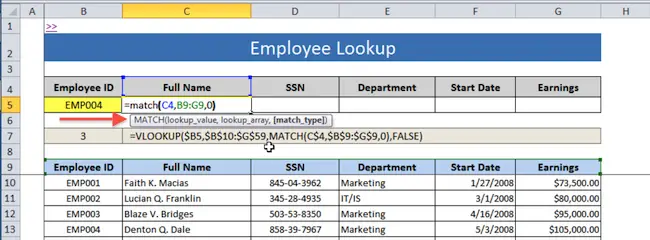We build up the VLOOKUP + MATCH formula in steps. The first step is to write

the MATCH function and check that it works correctly.

The formula above gives a value of 2, which is correct, because the first column (“Employee ID”) does not contain “Full Name”, whereas the second column does contain “Full Name”.

I will modify the MATCH formula to make the range B9:G9 into absolute references by selecting it and pressing F4. The reason is that we want to copy this across and have it update with the correct range, so copy the formula using CONTROL + C, then paste it to the cells on the right, pressing CONTROL + ALT + V to paste special as formulas.

When the formula is copied across to the right, you can see the results are 2, 3, 4, 5 and 6. What it’s done is evaluates the correct column number to use in our lookup formula by matching the contents of row 4 to the contents of row 9.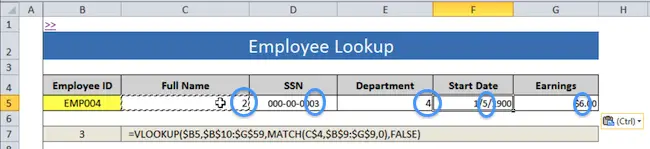You can see that copying across the MATCH formula results in the right column

number being calculated.

## Time to combine the MATCH function and VLOOKUP function

Now we now work through the VLOOKUP formula to see why the MATCH function can be very useful.

This is the combined VLOOKUP + MATCH formula: “=VLOOKUP(\$B\$5, \$B\$10:\$G\$59, MATCH(C\$4, \$B\$9:\$G\$9, 0), FALSE)

For the third function argument column_index, we use the MATCH function to lookup up the value “Full Name” in cell C4, and find its position in the range of column headings in B9:G9.

Note the use of absolute references to lock the first argument of MATCH lookup_value to row 4, and lock the second argument of MATCH lookup_array to B9:G9. Set the match_type to 0 for exact match.

Copy the VLOOKUP + MATCH formula from cell C5 using the keyboard combination CONTROL + C, then paste special as formulas into cells D5:G5 using the keyboard combination CONTROL + ALT + V for the paste special dialog box.

This is the result: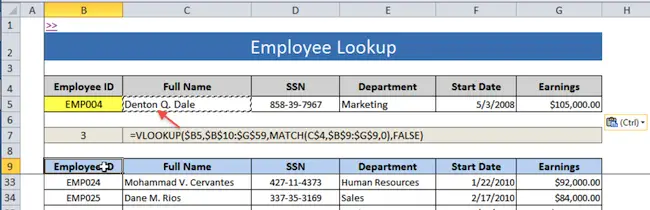We have completed the VLOOKUP + MATCH formula and copied it across to all cells in

our lookup table. Note that if you change the values in row 4, the VLOOKUP formulas in

row 5 dynamically update to lookup up the correct column.

You will see that by writing one VLOOKUP + MATCH formula and copying it across, you did not need to manually change the col_index_num inside the VLOOKUP formula. And if you were to change the value to cell C4 from “Full Name” to “Department”, the VLOOKUP formula in cell C5 dynamically knows to look up the “Department” instead of the “Full Name”. This demonstrates the flexibility of the VLOOKUP + MATCH combination.

## What could go wrong with Dynamic VLOOKUPs?

Here’s a brief list of things that could go wrong with your dynamic VLOOKUP formulas. It’s by no means a comprehensive list, but covers a few fundamental errors:

• Using COLUMNS function (Method 2) when the order of your lookup table headers does not match the order of your data table headers (e.g. “Full Name, SSN, Department, Start Date” vs. “Full Name, Department, SSN, Start Date”)
• Your absolute references might not be correct, and as you copy your dynamic VLOOKUP formula into other cells the COLUMNS() or MATCH() function could be looking up the wrong cells.

## Time for you to practise your VLOOKUP formulas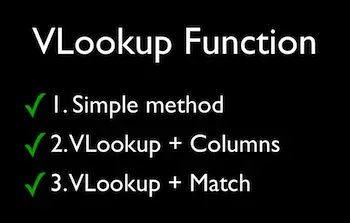I recommend you go to Excel and play around with VLOOKUP functions and try to insert COLUMN and MATCH to make the VLOOKUP formulas dynamic, because that way, you’ll master them quicker. All right? Have fun!
If you found the video and article helpful share it with three or more of your colleagues and friends using the sharing buttons on this page, or email

### Related Posts:Author: Victor Chan

Victor expertly teaches Microsoft Excel to people all over the world. He has millions of views of his popular Excel explainer videos on YouTube. These show time-saving shortcuts and real-world applications explained with easy-to-follow visuals.

Victor has over 20 years of experience using Excel as a professional for Big 4 Audit Firm Deloitte and two global tech companies. He knows firsthand that being more productive with Excel can lead to greater job satisfaction and career growth.

Recommended Posts

Discover the PROVEN Blueprint for transforming your Excel skills, supercharging your productivity, and standing out in your career! My course helps you to learn Excel VBA and save hours of time even if you have zero prior experience with programming.

Solve tricky Excel problems and take your work to the next level! Get customized solutions for your unique needs. Save time and gain insights with truly expert Excel solutions from only \$97 per task.

Get a clear overview of your project progress using the Excel project timeline. Use it to communicate the big picture, track task progress, and stay on top of your project goals. Stay organized with our project timeline!

Our cheat sheets provide quick and easy reference to commonly used Excel VBA concepts and code snippets.

Unlock new levels of productivity and efficiency with our cheat sheets, and write VBA code like a pro in no time.